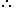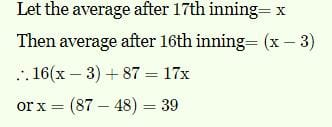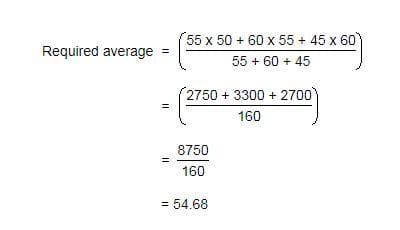# Practice Test: Averages - 2

## 10 Questions MCQ Test Quantitative Reasoning for GMAT | Practice Test: Averages - 2

Description
Attempt Practice Test: Averages - 2 | 10 questions in 20 minutes | Mock test for Quant preparation | Free important questions MCQ to study Quantitative Reasoning for GMAT for Quant Exam | Download free PDF with solutions
QUESTION: 1

### A grocer has a sale of Rs. 6435, Rs. 6927, Rs. 6855, Rs. 7230 and Rs. 6562 for 5 consecutive months. How much sale must he have in the sixth month so that he gets an average sale of Rs. 6500?

Solution:

Total sale for 5 months = Rs. (6435 + 6927 + 6855 + 7230 + 6562) = Rs. 34009.Required sale = Rs. [ (6500 x 6) - 34009 ]

= Rs. (39000 - 34009)

= Rs. 4991.

QUESTION: 2

### A batsman makes a score of 87 runs in the 17th inning and thus increases his averages by 3. What is his average after 17th inning?

Solution:QUESTION: 3

### Arun obtained 76, 65, 82, 67 and 85 marks (out in 100) in English, Mathematics, Chemistry, Biology and Physics. What is his average mark?

Solution:

Average = (76 + 65 + 82 + 67 + 85 )/ 5 = 375/5 =  75.

QUESTION: 4

If the average marks of three batches of 55, 60 and 45 students respectively is 50, 55, 60, what is the average marks of all the students?

Solution:QUESTION: 5

The average age of husband, wife and their child 3 years ago was 27 years and that of wife and the child 5 years ago was 20 years. What is the present age of the husband?

Solution:

Sum of the present ages of husband, wife and child = (27 * 3 + 3 * 3) years = 90 years.
Sum of the present ages of wife and child = (20 * 2 + 5 * 2) years = 50 years.
Husband's present age = (90 - 50) years
= 40 years

QUESTION: 6

The average weight of 8 person's increases by 2.5 kg when a new person comes in place of one of them weighing 65 kg. What is the weight of the new person?

Solution:

The average weight of 8 persons increases by 2.5 kg
Total weight of 8 persons increased = 8 × 2.5 kg = 20 kg.
New person comes in place of one of them weighing 65 kg.
Hence the increase of weight of 8 persons together is because of new person replacing the person having 65 kg weight. Accordingly
Weight of new person = 65 + 20 kg = 85 kg
Thus the person replacing the 65 kg person should have 85 kg weight, which causes increase of weight of the group together by 2.5 kg .

QUESTION: 7

The average weight of A, B and C is 45 kg. If the average weight of A and B be 40 kg and that of B and C be 43 kg, what is the weight of B?

Solution:

Let A, B, C represent their respective weights. Then, we have:

A + B + C = (45 x 3) = 135 .... (i)

A + B = (40 x 2) = 80 .... (ii)

B + C = (43 x 2) = 86 ....(iii)

Adding (ii) and (iii), we get: A + 2B + C = 166 .... (iv)

Subtracting (i) from (iv), we get : B = 31.B's weight = 31 kg.

QUESTION: 8

A student needed to find the arithmetic mean of the numbers 3, 11, 7, 9, 15, 13, 8, 19, 17, 21, 14 and x. He found the mean to be 12. What is the value of x?

Solution:

As, Mean = Total Sum of Observations / No. of Observations

Clearly, we have (3 + 11 + 7 + 9 + 15 + 13 + 8 + 19 + 17 + 21 + 14 + x) / 12 = 12.

or  137 + x = 144

⇒ x = 144 - 137 = 7.

QUESTION: 9

There are two divisions A and B of a class, consisting of 36 and 44 students respectively. If the average weight of divisions A is 40 kg and that of division b is 35 kg. What is the average weight of the whole class?

Solution:
QUESTION: 10

Distance between two stations A and B is 778 km. A train covers the journey from A to B at 84 km per hour and returns back to A with a uniform speed of 56km per hour. Find the average speed of the train during the whole journey?

Solution:

Avg speed of train = 2xy/x+y
⇒ [2(84)(56)]/(84+56)
⇒ [2(84)(56)]/140
= 67.2km/hrUse Code STAYHOME200 and get INR 200 additional OFF Use Coupon Code